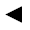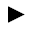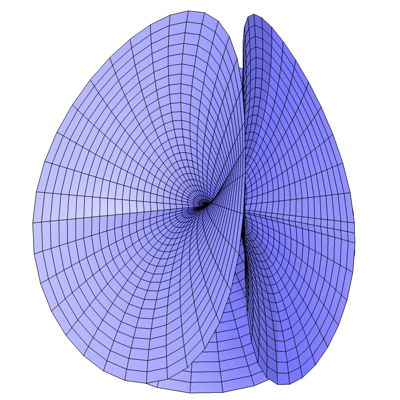[back]

## 3.31. Maeder's Owl

[next]The Maeder's Owl [ 8 ] is represented by the following equations.

 x = v cos(u) - 0.5 v 2 cos(2 u) 3-108 y = -v sin(u) - 0.5 v 2 sin(2 u) 3-109 z = 4exp(1.5log(v))cos(3u/2)/3 3-110

To represent the area, the two parameters u and v can assume the following values ​​(domain of definition), for example.

 u is an element from the set of numbers [0, 4 pi] v is an element of the number set [0.001, 1]

Maeder's Owl is a semi-open figure, so the domain of v can be changed in the plugin.Fig. 35